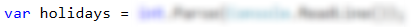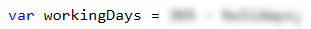# Problem: Sleepy Tom Cat

Tom Cat likes to sleep all day but, unfortunately, his owner is always playing with him whenever he has free time. In order to sleep well, the norm of games that Tom has is 30 000 minutes per year. The time for games he has depends on the holidays that his owner has:

• During work days, his owner plays with him 63 minutes per day.
• During holidays, his owner plays with him 127 minutes per day.

Write a program that reads the number of holidays and prints whether Tom can sleep well and how much the difference from the norm for the current year is. It is assumed that there are 365 days in one year.

Example: 20 holidays -> the working days are 345 (365 - 20 = 345). The time for games is 24 275 minutes (345 * 63 + 20 * 127). The difference from the norm is 5 725 minutes (30 000 – 24 275 = 5 725) or 95 hours and 25 minutes.

## Input Data

The input is read from the console and consists of an integer – the number of holidays in the range of [0 … 365].

## Output Data

Two lines have to be printed on the console:

• If Tom's time for games is above the norm for the current year:
• On the first line print: “Tom will run away”
• On the second line print the difference from the norm in the format:
“{H} hours and {M} minutes more for play”
• If the time for games of Tom is below the norm for the current year:
• On the first line print: “Tom sleeps well”
• On the second line print the difference from the norm in the format:
“{H} hours and {M} minutes less for play”

## Sample Input and Output

Input Output Input Output
20 Tom sleeps well
95 hours and 25 minutes less for play
113 Tom will run away
3 hours and 47 minutes for play

## Hints and Guidelines

In order to solve the problem, we will read the input data. Then, we will write a few conditional statements and do some calculations. Finally, we will print the result.

From the task we see that the input data will be read only on the first line and will be an integer in the range of [0 … 365]. This is why we will use a variable of int type.### Calculating Working Days

To solve the problem, first we have to calculate the total minutes the owner of Tom is playing with him. We see that not only does the sleepy cat has to play with his owner during the holidays, but also during the working days. The number that we read from the console refers to the holidays.

Out next step is to calculate, with the help of that number, how many the working days of the owner are, as without them we cannot calculate the total minutes for play. As the total number of days per year is 365 and the number of holidays is X, that means that the number of working days is 365 - X*. We store the difference in a new variable that only stores this value.### Calculating Playing Time

Once we have the number of days for playing, we can calculate the time for games of Tom in minutes. Its value is equal to the result of the multiplication of the working days by 63 minutes (the task specifies that during working days, the time for play is 63 minutes per day), summed with the result of the multiplication of the holidays by 127 minutes (the task specifies that during holidays, the time for play is 127 minutes per day).In the task condition we see that we have to print the difference between the two values in hours and minutes as output data. That is why we subtract the total time for play from the norm of 30 000 minutes and store the result in a new variable. After that, we divide that variable by 60 to get the hours, and then, to find out how many the minutes are, we use modular division with the operator %, as again we divide the variable of the difference by 60.

Here we have to note that if the total time for play of Tom is less than 30,000, when subtracting the norm from it, we will obtain a negative number. In order to neutralize the number in the division, we use the method Math.Abs(…) when finding the difference.### Checking the Conditions

The time for games is already calculated, which leads us to the next step – comparing the time for play of Tom with the norm on which the good sleep of the cat depends. For that we will use an if-else conditional statement. In the if clause we will check whether the time for play is more than 30 000 (the norm).

### Processing the Output Data

Whatever the result of the conditional statement is, we have to print how much the difference in hours and minutes is. We will do that with a placeholder and the variables that store the values of the hours and the minutes, as the formatting will be according to the task requirements for output.